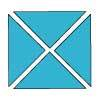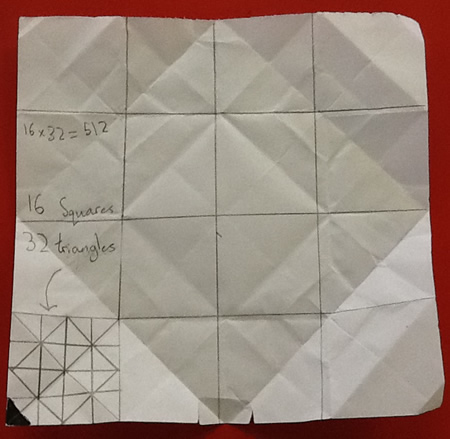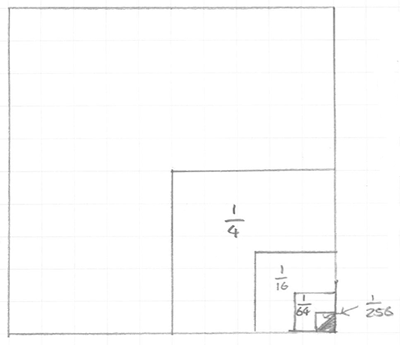#### You may also like### Chocolate

There are three tables in a room with blocks of chocolate on each. Where would be the best place for each child in the class to sit if they came in one at a time?### Four Triangles Puzzle

Cut four triangles from a square as shown in the picture. How many different shapes can you make by fitting the four triangles back together?### Cut it Out

Can you dissect an equilateral triangle into 6 smaller ones? What number of smaller equilateral triangles is it NOT possible to dissect a larger equilateral triangle into?

# Bryony's Triangle

##### Age 7 to 11Challenge Level

Izak, who is home schooled, told us how he went about tackling this problem:

After folding the flower and shading the triangle, I got another square of paper the same size as the first one, but left it unfolded. I traced the shaded triangle onto the corner of the unfolded square of paper.
Then, I divided the unfolded square into $16$ equal squares.
Then, I used a ruler to divide one of the $16$ squares into triangles the same size as the shaded one on the flower. I counted the number of triangles in the $16$th of the square. There were $32$ total triangles in that $16$th.

$32 \times 16 = 512$

So, there are $512$ triangles the size of the shaded one in the original square of paper, therefore the shaded triangle is $\frac{1}{512}$.

Well done also to Josh from Goonhavern whose solution was similar to Izak's.  Josh sent us this photo:Nikki C and Dominic B from Woore Primary School, and AI and JB from Gledhow Primary, used a similar method.  Here is what AI and JB had to say:

The solution to the question is $\frac{1}{512}$.
The explanation is, after we created the flower we marked the triangle as we needed to find out what fraction this triangle was out of the original square.
We unfolded the flower so we had the original square with the marked triangle in the bottom corner.
We marked out $\frac{1}{4}$ of the square, we then quartered this which gave us $\frac{1}{16}$.
We quartered again and got $\frac{1}{64}$.
Yet again we quartered giving us $\frac{1}{256}$.
The marked triangle was half of this so this time we halved and got $\frac{1}{512}$.

Mark, also from Gledhow Primary, used the same approach and he sent us a picture to help us see what he did:Joe, Sam, Ollie and Matthew from Keer Mackie Primary used another slightly different method:

First we worked out there were $16$ squares in the paper.
Next we worked out that in the squares were $4$ triangles so we found the product of $4$ and $16$ which was $64$.
Our next step was to find out how many of the smallest triangles fitted in the biggest triangle.  The answer was $8$ so we timesed $8$ by $64$ and our final answer was $512$.

Ellie from Wellesley Park had a good way of using trial and improvement to solve this challenge:

I made the flower then opened it up and counted all the triangles. I counted 16. I worked out what each triangle represented. I cut out a triangle and tried to fit it into the small triangle. I kept trying until the triangle fitted perfectly onto the sheet of paper. Finally, the triangle fitted. The fraction is $\frac{1}{512}$.

Thank you for your clear solutions.  It's not easy explaining how you worked on a problem when it involves folding paper!